# Parsing and generating ABIF files (DNA chromatograms)

Posted on January 9, 2019

# Introduction

Hyrax ABIF is a Haskell package, that I created at HyraxBio to test our bioinformatics software pipeline. We have released the HyraxAbif package as open source (BSD3 licence) in the hopes that it will be useful to others.

In this post I’ll show how the package can be used as a standalone tool as well as looking at how the Haskell code works. Skip directly to the usage / ABIF format / Haskell sections if that is all you are interested in reading about.

# Licence

See the LICENCE file. Please note that this package is distributed without warranties or conditions of any kind.

# Chromatograms & some basic biology

## Chromatograms

Part of what we do at HyraxBio is analyze DNA sequences to determine drug resistance for various pathogens. The first step in this process getting DNA data from a sequencing machine. The mechanics of sequencing are pretty complex. Fortunately for us we start with the data already sequenced which means that all the “wet-work” is done and we can analyse and interpret the results as data, i.e. bioinformatics.

DNA is made up of four bases `A`, `C`, `G` and `T` (adenine, cytosine, guanine, and thymine respectively). A sequencing machine takes DNA strands and determines the sequences of bases that are present. There is a fair amount of complexity here. You can’t simply grab a strand of DNA and read it in its entirety and certainly not with 100% accuracy (because biology). Rather the DNA is amplified and a consensus of reads for each position in the DNA strand is calculated. There can be both (many) variations of the same virus (mutations) as well as errors in the reading process itself. So each position is calculated based on which of the bases have the strongest signal per position.

ABIF files are generated by these sequencing machines by using chemical reactions that release a tiny amount of coloured light when a reagent reacts with one of the bases. Each base results in a different colour which enables the machine to detect which base is present DNA. The details behind this are fascinating see e.g. wikipedia for more detail if you are interested.

Below is a section of a chromatogram showing a wave for each of the four bases.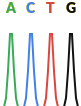This is a perfect chromatogram, there are often multiple possibilities per position of different intensity. In the image below you can see that the second and third positions have more than one possible base, this is called a mix.Even that is an unnaturally clean chromatogram. In reality they often look more like this, and take complicated base calling software and/or trained lab workers (or overworked PHD students) to decide on what base is actually represented.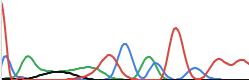The chromatogram data in ABIF format is fed into base calling software like PHRED and/or recall which analyze the chromatogram and decide on which base to call per position. The result being a string of bases (A/C/G/T).

## Why we created hyraxAbif

Testing a full bioinformatics pipeline is critical to ensuring that every step works correctly and results in high quality outputs. The problem is that we could find no practical existing way to generate our own chromatograms (ABIFs). It is possible to use a set of existing ABIF files but this has two major problems

• These are DNA sequences from real people so there are confidentiality issues
• More practically, we needed very specific input data to test decisions further down the pipeline and finding real data with the exact mutations and no others is not realistic.

HyraxABIF was created to resolve this. It lets us easily create chromatograms from a DNA sequence and thus do all the testing we need to.

## A bit more biology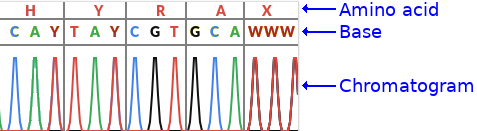The image above has a bit more detail, it shows the bases including ambiguous ones as well as the amino acids.

### Ambiguous bases

As discussed above there could be multiple possibilities per position. The IUPAC ambiguity codes (see wikipedia ) or bioinformatics.org are a way of encoding the ambiguity in a single letter. For example a `Y` IUPAC code means that the base is either a `C` or a `T`.

### Amino acids

Each group of three nucleotide bases is called a codon and encodes for a single amino acid (in coding regions…). The chromatogram also shows the amino acid per codon. This is not important to know for this post but may help if you see other pictures of other chromatograms as this will usually be show.

# Using the application

HyraxAbif’s primary goal was for generating chromatograms from an input DNA sequence. It can be installed from hackage with cabal, from stack, or by cloning the git repo.

## Generating a simple ABIF

The input for generating a chromatogram is a simplified FASTA format file. These files look like this

``````> 1
ACTG``````

The first line is the weight (more on this later), the second is the DNA sequence. Given this input file you would run

`hyraxAbif-exe gen inputDir/ outputDir/`

and you would end up with a ABIF file and a chromatogram like thisFor many scenarios this is all you’ll need. You create a folder of FASTA input files and get a folder of generated corresponding ABIF files.

### Generating more complex chromatograms

You can also generate chromatograms with mixes. The first line has the weight for the sequence, and each FASTA file can contain multiple reads.

``````> weight
> weight
• The weight is a numeric value between 0 and 1 that specifies the weight of the current read i.e. the intensity of the peak.
• No other header/name is allowed (no quality data / naming etc)
• The read is the set of input nucleotides, IUPAC ambiguity codes are supported (MRWSYKVHDBNX).
• A read can be single or multi-line
• Weights for each position are summed to a maximum of 1.0 per nucleotide
• You can use _ as a “blank” nucleotide, in which case only the nucleotides from other reads will be considered
• Reads need not be the same length

For example

``````> 0.5
ACG
> 0.3
AAAA
> 1
__AC``````

Results in the following weighted nucleotide per position

position A C G T
0 0.5 + 0.3 = 0.8 0 0 0
1 0.3 0.5 0 0
2 0.3 + 1 = 1.0 0 0.5 0
3 0.3 1 0 0

Note that 0.3 + 1.0 = 1.0 because the max value is 1.0

And this sample

``````> 1
ACAG
> 0.3
_GT
> 0.2
_G``````

results in this chromatogram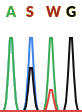The IUPAC codes here are

• `S` = `G` or `C`
• `W` = `A` or `T`

A weighted FASTA can represent a reverse read. To do this add a `R` suffix to the weight. The data you enter should be entered as if it was a forward read. This data will be complemented and reversed before writing to the ABIF

``````> 0.9R
ACAG``````

which results in the sequence `TGTC`

## Dumping an existing ABIF file

You can also dump an existing ABIF file

`hyraxAbif-exe dump sample.abif`

This prints two views of the file. First a detail view, partially show belowand then a summary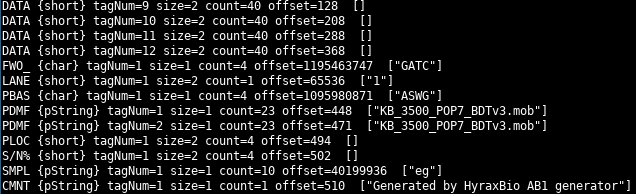For certain types of data (e.g. strings) the parsed value is displayed.

# The ABIF file format

The ABIF format is documented here. As you can see from the spec the ABIF format was modeled after the TIFF format. This means that there is a directory of entries and each entry has a data type.

The spec is quite thorough and explains the layout well. If you are wanting to understand the format it is your best starting point. The spec, however, only goes into detail on the ABIF structure. It does not go into much detail on how the chromatogram data itself is stored, I’ll cover that here.

In the file after the ABIF header and version number is the root directory entry. This entry points to the first of the data directory entries that can be located at any other location in the file.

Each directory entry has an offset to the location of its data in the file, the data size, the element size and number of entries. See the spec or the discussion of the Haskell code below for more details on each field.

Note that for data with a size of four bytes or less, the data is stored in the offset field itself.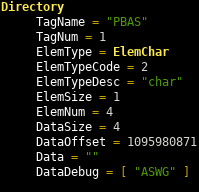## Important ABIF directory entries

Towards the end of the spec are examples of the layout of ABIF files for a few sequencing machines. Lets take a look at some of these for the 3500 layout.

### Chromatogram traces

Type Tag Number Contains
DATA 1 - 4 The raw data per base (channel). The order of the bases is specified by the FWO_ entry
DATA 5 Short Array holding measured volts/10 (EP voltage) during run
DATA 6 Short Array holding measured milliAmps trace (EP current) during run
DATA 7 Short Array holding measured milliWatts trace (Laser EP Power) during
DATA 8 Short Array holding measured oven Temperature (polymer temperature) trace during run
DATA 9 - 12 Short Array holding analyzed color data

This is a pretty intimidating set of values we thought we would have to generate from a FASTA input, just for the traces. Fortunately through trial and error we were able to see that only a small subset of the entries were required for the base calling software we were using (PHRED + Recall). All we needed to generate were the data sections 9 to 12, i.e. one per base, the analyzed colour data.

The four DATA sections we need to generate (entries 9 through 12) contain an array of shorts. Each short represents the intensity of the light for that base at a given point. Each of these DATA sections have the wave of the light intensity over time. The FWO_ directory entry specifies which base each DATA entry represents. We always generate it in the 3500 format, so the order is `9=G`, `10=A`, `11=T`, `12=C`.

### Peak location

The traces above are just the wave form, the PLOC entry specifies the location of each peak. This is the location, across all four DATA entries, where the peak of the waves should be found. There is a single PLOC entry for all four DATA entries.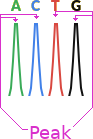### Other required entries

The other required entries are easy to generate, they are things like the base order (FWO_), file name (PDMF) and called based (PBAS). See the Haskell code discussion below to see them all.

### Generating the waveforms

Given a base, we then need to create a wave and a single peak location entry. The data we use for each wave is this array of shorts `[0, 0, 128, 512, 1024, 1024, 512, 128, 0, 0]` which creates a wave like this.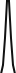The peak is the middle of the wave, nice and simple.

Again see the code discussion for more details in this.

# Using the code, some basic examples

## Reading and printing the ABIF structure

``````{-# LANGUAGE NoImplicitPrelude #-}

import           Protolude

-- | Read and print a ABIF file

case abif' of
Left e -> putStrLn \$ "error reading ABIF: " <> e
Right abif ->
-- Print after removing the data, to make it readable
print \$ H.clearAbif abif``````
1. `readAbif` tries to parse an ABIF file, it returns an `Either Text Abif`
2. Check if the file was parsed successfully
3. If not (Left) then print the error
4. If successful (Right) then
1. `clearAbif` removes all the raw data. If you don’t do this then all the massive byte arrays will get printed too
2. `print` the result.

The functions are all commented and visible on hackage.

## Adding a comment to an existing ABIF

``````{-# LANGUAGE NoImplicitPrelude #-}

import           Protolude
import qualified Hyrax.Abif.Write as H

-- | Add a comment to an existing AB1 file

case abif' of
Left e -> putStrLn \$ "error reading ABIF: " <> e
Right abif -> do
let modified = H.addDirectory abif \$ H.mkComment "new comment"
H.writeAbif "example.modified.ab1" modified``````

Only the Right case is different than the previous example

1. `addDirectory` is called to add a new comment directory entry that is created by `mkComment`
2. `writeAbif` writes the updated file to disk

## More examples

The `Examples` directory contains more examples. You can also look at the `Generate` and `Main` modules to see how the code is used.

# Understanding the code

## Data.Binary

Data.Binary is used to read and write the raw bytes in the ABIF files.

### Writing

Below is an example of writing two Int8 and an Int32 value.

``````{-# LANGUAGE NoImplicitPrelude #-}

module Main where
import Protolude
import qualified Data.Binary.Put as B
import qualified Data.ByteString.Lazy as BSL

main :: IO ()
main = do
let outData = B.runPut testWrite
BSL.writeFile "test.dat" outData

where
testWrite :: B.PutM ()
testWrite = do
B.putInt8 1
B.putInt8 2
B.putInt32be 3``````

`runPut` “runs” the `PutM` monad. `testWrite` can then simply call the `put*` functions to write the data in whatever format is required.

This creates a file that looks like this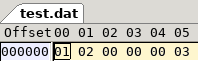Reading the data from the file looks like this

``````{-# LANGUAGE NoImplicitPrelude #-}

module Main where
import Protolude
import qualified Data.Binary.Get as B
import qualified Data.ByteString.Lazy as BSL

main :: IO ()
main = do

where
testRead :: B.Get (Int8, Int8, Int32)
a <- B.getInt8
b <- B.getInt8
c <- B.getInt32be
pure (a, b, c)``````

`runGet` is given the ByteString from the file and `testRead` gets the values in the appropriate format.

If you prefer applicatives you could instead have written `testGet` as

``(,,) <\$> B.getInt8 <*> B.getInt8 <*> B.getInt32be``

and `testWrite` as

``B.putInt8 1 *> B.putInt8 2 *> B.putInt32be 3``

## ABIF Types

Hyrax.Abif contains the core types for the package

###### src/Hyrax/Abif.hs (22 to 32)
``````module Hyrax.Abif
( Abif (..)
, Directory (..)
, ElemType (..)
, getElemType
, describeElemType
) where

import           Protolude
import qualified Data.ByteString.Lazy as BSL``````
###### src/Hyrax/Abif.hs (37 to 64)
``````-- | A single ABIF
, aRootDir :: !Directory
} deriving (Show, Eq)

, hVersion :: !Int
} deriving (Show, Eq)

-- | ABIF directory entry.
-- The 'dData' field contains the data for the entry
data Directory = Directory { dTagName :: !Text        -- ^ Tag name
, dTagNum :: !Int          -- ^ Tag number, see e.g. how DATA entries use this
, dElemType :: !ElemType   -- ^ Type of an element
, dElemTypeCode :: !Int    -- ^ Integer value of 'dElemType'
, dElemTypeDesc :: !Text   -- ^ Description of 'dElemType'
, dElemSize :: !Int        -- ^ Size in bytes of each element
, dElemNum :: !Int         -- ^ Number of elements in the data. See the spec per data type. E.g. for a string this is the number of characters
, dDataSize :: !Int        -- ^ Number of bytes in the data
, dDataOffset :: !Int      -- ^ Offset of this directory entry's data in the file. For data that is four
--    bytes or less, the data itself is stored in this field.
--    This value will be recalculated when writing an ABIF so you do not need to manually set it.
, dData :: !BSL.ByteString -- ^ The entry's data
, dDataDebug :: ![Text]    -- ^ Optinal debug data, populated by 'Hyrax.Abif.Read.getDebug' when a ABIF is parsed
} deriving (Show, Eq)``````

These three types make up most of what we need to represent an ABIF. A few things to notice

• The root directory in the `Abif` type will point to the array of `Directory` entries
• `dElemTypeCode` is the integer value read from the file (see the spec for the codes). `dElemType` and `dElemTypeDesc` are interpreted values from this
• `dElemSize` and `dElemOffset` are read from the file, but are automatically calculated when writing (see the Hyrax.Abif.Write section below)
• `dData` is the actual raw data read from the file, or data to be written to an ABIF file
• `dDataDebug` is populated while reading the file and used during dumping to give human readable info about the file being inspected.

### Element types

The remaining code is the definition of `ElemType` and functions for interpreting the raw element type integer value. Note that the spec defines a number of unsupported data types, these are included here.

`readAbif` calls `getAbif` to parse the data

``````-- | Read and parse an AB1 file
readAbif :: FilePath -> IO (Either Text Abif)

`getAbif` starts the parsing of the `Abif` data structure.

``````-- | Parse an AB1 from a 'ByteString'
getAbif :: BSL.ByteString -> Either Text Abif
getAbif bs = do
(header, rootDir) <- case B.runGetOrFail (getRoot bs) bs of
Right (_, _, x) -> pure x
Left (_, _, e) -> Left ("Error reading root: " <> Txt.pack e)

let dirBytes = BSL.drop (fromIntegral \$ dDataOffset rootDir) bs

ds <- case B.runGetOrFail (getDirectories bs [] \$ dElemNum rootDir) dirBytes of
Right (_, _, x) -> pure x
Left (_, _, e) -> Left ("Error reading "
<> show (dElemNum rootDir)
<> " directories (at " <> show (dDataOffset rootDir) <> "): "
<> Txt.pack e
)

pure \$ Abif header rootDir ds``````
• The `Either` monad is used so any `Left` value will short-circuit out of the function and return the `Left` value immediately.
• `Data.Binary.runGetOrFail` returns a `Left` if the get operation fails. Much better than getting an exception as you would with `runGet`
• The first step is to read the header and the root directory
• Then the ABIF directory entries are read. These directories are found at the offset specified in the root directory. The code “goes” to this offset by dropping the number of bytes from the read data.
• `getDirectories` reads the number of directory entries specified by the root entry.

`getRoot` gets the header and root directory (see next section)

``````-- | Parse the root ('Header' and 'Directory')
getRoot :: BSL.ByteString -> B.Get (Header, Directory)
getRoot bs = do
rd <- getDirectory bs
pure (h, rd)``````

`getHeader` gets the “ABIF” magic string and version number. Similar to the read example above.

``````-- | Parse the ABIF 'Header'
Header <\$> (TxtE.decodeUtf8 <\$> B.getByteString 4)
<*> (fromIntegral <\$> B.getInt16be)``````

`getDirectories` is given the number of directories to read. It tries to read a single `Directory` by calling `getDirectory` and then recursively calls itself until done.

``````-- | Parse all the directoy entries
getDirectories :: BSL.ByteString -> [Directory] -> Int -> B.Get [Directory]
getDirectories _ acc 0 = pure acc
getDirectories bs acc more = do
d <- getDirectory bs
B.skip 4 -- Skip the reserved field
getDirectories bs (acc <> [d]) (more - 1)
``````

Reading and individual directory is done by `getDirectory`.

• Read the values and convert into appropriate types (e.g. Int8 to Int)
• If the data is four bytes or less then the offset field contains the data
• If the data is larger than four bytes, go to the offset and read the entire chunk as a `ByteString`.
• Create a `Abif` value
``````-- | Parse a single 'Directory' entry and read its data
getDirectory :: BSL.ByteString -> B.Get Directory
getDirectory bs = do
tagName <- TxtE.decodeUtf8 <\$> B.getByteString 4
tagNum <- fromIntegral <\$> B.getInt32be
typeCode <- fromIntegral <\$> B.getInt16be
elemSize <- fromIntegral <\$> B.getInt16be
elemNum <- fromIntegral <\$> B.getInt32be
dataSize <- fromIntegral <\$> B.getInt32be
offsetDataBytes <- B.lookAhead \$ B.getLazyByteString 4
dataOffset <- fromIntegral <\$> B.getInt32be

--  Data that is 4 bytes or less is stored in the offset field
dataBytes <- if dataSize <= 4
then pure \$ BSL.take (fromIntegral dataSize) offsetDataBytes
else case B.runGetOrFail (B.getLazyByteString \$ fromIntegral dataSize) \$ BSL.drop (fromIntegral dataOffset) bs of
Right (_, _, x) -> pure x
Left (_, _, e) -> fail \$ "error reading data ("
<> show dataSize
<> " bytes starting at "
<> show dataOffset
<> ") for directory entry '"
<> Txt.unpack tagName
<> "': "
<> e

let (elemType, elemCode) = describeElemType typeCode
pure Directory { dTagName = tagName
, dTagNum = tagNum
, dElemTypeCode = typeCode
, dElemTypeDesc = elemCode
, dElemType = elemType
, dElemSize = elemSize
, dElemNum = elemNum
, dDataSize = dataSize
, dDataOffset = dataOffset
, dData = dataBytes
} ``````

• A `PString` is prefixed with an `Int8` size. So read the size and then the string
• A `CString` is null terminated, so read all the data for the length of the string (from the directory entry) and drop the final null character.
``````-- | Parse a 'ElemPString'
getPString :: B.Get Text
getPString = do
sz <- fromIntegral <\$> B.getInt8
TxtE.decodeUtf8 <\$> B.label ("PString length=" <> show sz <> ".") (B.getByteString sz)

-- | Parse a 'ElemCString'
getCString :: Int -> B.Get Text
getCString sz =
TxtE.decodeUtf8 <\$> B.getByteString (sz - 1)``````

### Debug info

getDebug adds human readable information for some types, e.g. for strings. This lets us print the `Abif` structure to the console with some useful data. Only a portion of `getDebug` is show here as it is a little repetitive. However it is a good function to look at to see more examples of reading the raw data.

``````-- | Populate the directory entry with debug data (into 'dDataDebug').
-- This is done for selected types only, e.g. for strings so that printing the structure will display
getDebug :: Directory -> Directory
getDebug d =
let bsAtOffset = dData d in

case dElemType d of
-- Strings have a count = number of chars, not number of "strings"
ElemPString ->
if dDataSize d <= 4
then d { dDataDebug = [TxtE.decodeUtf8 . BSL.toStrict . BSL.drop 1 . BSL.take (fromIntegral \$ dDataSize d) \$ dData d] }
else d { dDataDebug = [B.runGet (lbl getPString) bsAtOffset] }

-- Strings have a count = number of chars, not number of "strings"
ElemCString ->
if dDataSize d <= 4
then d { dDataDebug = [TxtE.decodeUtf8 . BSL.toStrict . BSL.take (fromIntegral \$ dDataSize d - 1) \$ dData d] }
else d { dDataDebug = [B.runGet (lbl . getCString \$ dDataSize d) bsAtOffset] }``````

When printing the structure it does not make sense to print all the raw data too. So the `clear*` functions remove that before printing

``````-- | Removes all data from the ABIF's directories
clearAbif :: Abif -> Abif
clearAbif a = a { aRootDir = clear \$ aRootDir a
}

-- | Removes all data from a directory entry. This will probably only be useful when trying to show an ABIF value
clear :: Directory -> Directory
clear d = d { dData = "" }``````

## Hyrax.Abif.Write

As with the read functions there are two write functions for writing to `ByteString` or to a file.

###### src/Hyrax/Abif/Write.hs (54 to 64)
``````-- | Write an 'Abif' to a 'ByteString'
createAbifBytes :: Abif -> BSL.ByteString
createAbifBytes ab1 =
B.runPut (putAbif ab1)

-- | Write an 'Abif' to a file
writeAbif :: FilePath -> Abif -> IO ()
writeAbif destPath ab1 = do
let b = createAbifBytes ab1
BS.writeFile destPath \$ BSL.toStrict b``````

Writing the ABIF data is relatively simple since each directory entry already contains the `ByteString` raw data. `putAbif` does need to recalculate the data size though

###### src/Hyrax/Abif/Write.hs (69 to 98)
``````-- | Create the 'Abif' using "Data.Binary"
putAbif :: Abif -> B.Put
putAbif (Abif header root dirs) = do
-- Total data size
let dataSize = foldl' (\acc i -> if i > 4 then acc + i else acc) 0 \$ dDataSize <\$> dirs

-- Data starts at offset 128
let startDataOffset = 128
-- Write the root directory entry
putDirectory (startDataOffset + dataSize) \$ root { dDataSize = 28 * length dirs
, dElemNum = length dirs
}

-- Write 47 zero Int16 values as required by the spec
traverse_ B.putInt16be \$ replicate 47 0
-- Write the data, for all data larger than four bytes. Data four bytes or less is stored
--  in the offset field
traverse_ (B.putLazyByteString . dData) \$ filter (\d -> dDataSize d > 4) dirs
-- Write the directory entries.
foldM_ writeDir startDataOffset dirs

where
writeDir offset dir = do
putDirectory offset dir
pure \$ if dDataSize dir > 4
then offset + dDataSize dir
else offset``````
• The total data size is calculated. It is the sum of all the non-root directory entries where the data is not stored in the offset field (i.e. where data size > 4 bytes)
• The data will starting being written at offset 128, i.e. immediately after the header and root directory entry
• Write the 47 zeros required by the spec
• Write all the data from each of the directory entries
• Write the directory entries, incrementing the offset for each entry with data > 4 bytes

Writing the header is pretty simple, write the magic string and version number.

###### src/Hyrax/Abif/Write.hs (117 to 121)
``````-- | Write a 'Header'
putTextStr \$ hName h
B.putInt16be . fromIntegral \$ hVersion h``````

### Strings

There are two functions for writing `Text` values

###### src/Hyrax/Abif/Write.hs (103 to 112)
``````-- | Write 'Text'
putTextStr :: Text -> B.Put
putTextStr t = B.putByteString \$ TxtE.encodeUtf8 t

-- | Write a 'ElemPString'
putPStr :: Text -> B.Put
putPStr t = do
B.putInt8 . fromIntegral \$ Txt.length t
B.putByteString \$ TxtE.encodeUtf8 t``````

### Directory

When writing a directory there a few things to take care of

• Ensure that the directory name is exactly 4 bytes long
• Write the offset for data > 4 bytes
• Write the data to the offset field if it is <= 4 bytes, ensure it is exactly 4 bytes long on disk
• Append the reserved zero value
###### src/Hyrax/Abif/Write.hs (126 to 142)
``````-- | Write a 'Directory'
putDirectory :: Int -> Directory -> B.Put
putDirectory dirOffset d = do
let name = Txt.justifyLeft 4 ' ' . Txt.take 4 \$ dTagName d
putTextStr name
B.putInt32be . fromIntegral \$ dTagNum d
B.putInt16be . fromIntegral \$ dElemTypeCode d
B.putInt16be . fromIntegral \$ dElemSize d
B.putInt32be . fromIntegral \$ dElemNum d
B.putInt32be . fromIntegral \$ dDataSize d

-- data with a size >= 4 are written in the offset
if dDataSize d > 4
then B.putInt32be . fromIntegral \$ dirOffset
else B.putLazyByteString . BSL.take 4 \$ dData d <> "\0\0\0\0"

B.putInt32be 0 -- reserved / datahandle``````

### mk* helper functions

The `mk*` set of functions help in constructing valid directory entries.

Below are two of these functions

• `mkBaseOrder` which creates a FWO_ Directory entry.
• `mkLane` which creates a LANE Directory entry.

As you can see these functions take appropriately typed values in and produce a valid directory entry for the data and directory type. (See `Hyrax.Abif.Generate` to see them in use)

###### src/Hyrax/Abif/Write.hs (48 to 49)
``````-- | Used to specify the base order for the FWO directry entry, see 'mkBaseOrder'
data Base = BaseA | BaseC | BaseG | BaseT``````
###### src/Hyrax/Abif/Write.hs (208 to 244)
``````-- | Create a base order (FWO_) 'Directory' entry data
mkBaseOrder :: Base -> Base -> Base -> Base -> Directory
mkBaseOrder w x y z =
Directory { dTagName = "FWO_" -- Base order
, dTagNum = 1
, dElemTypeCode = 2
, dElemTypeDesc = "char"
, dElemType = ElemChar
, dElemSize = 1
, dDataOffset = 0
, dData = getBase w <> getBase x <> getBase y <> getBase z
, dDataSize = 4
, dElemNum = 4
}
where
getBase BaseA = "A"
getBase BaseC = "C"
getBase BaseG = "G"
getBase BaseT = "T"

-- | Create a lane (LANE) 'Directory' entry and data
mkLane :: Int16 -> Directory
mkLane lane =
Directory { dTagName = "LANE" -- Lane or capliary number
, dTagNum = 1
, dElemTypeCode = 4
, dElemTypeDesc = "short"
, dElemType = ElemShort
, dElemSize = 2
, dElemNum = 1
, dDataSize = 2
, dDataOffset = 0
, dData = B.runPut \$ B.putInt16be lane
}``````

See the code or haddock for the full set of mk* functions.

`addDirectory` appends a directory entry to an existing `Abif`. See the examples to see this in use.

###### src/Hyrax/Abif/Write.hs (346 to 349)
``````-- | Add a directory to an 'Abif'
addDirectory :: Abif -> Directory -> Abif

## Hyrax.Abif.Generate and Hyrax.Abif.Fasta

Generating ABIFs is the main purpose of this package and the code to do this is in `Hyrax.Abif.Generate`. There is less than 200 lines of code, but I’ll go through how it works in some detail.

`generateAb1` is the main function in this module, it controls the flow of generating a single ABIF. It has the following high level concerns

1. Generate the traces per base from the weighted FASTA
2. Generate the peak locations
3. Generate the directories
4. Create the ABIF
###### src/Hyrax/Abif/Generate.hs (131 to 171)
``````-- | Create the 'ByteString' data for an AB1 given the data from a weighted FASTA (see 'readWeightedFasta')
generateAb1 :: (Text, [(Double, Text)]) -> BSL.ByteString
generateAb1 (fName, sourceFasta) =
let
tr = generateTraceData sourceFasta
valsPerBase = trValsPerBase tr
generatedFastaLen = (Txt.length \$ trFasta tr)

-- The point that is the peak of the trace, i.e. mid point of trace for a single base
midPeek = valsPerBase `div` 2
-- Get the peak locations for all bases
peakLocations = take generatedFastaLen [midPeek, valsPerBase + midPeek..]

-- Sample name (from the FASTA name)
sampleName = fst . Txt.breakOn "_" \$ fName

-- Create the ABIF directories
dirs = [ mkData  9 \$ trData09G tr -- G
, mkData 10 \$ trData10A tr -- A
, mkData 11 \$ trData11T tr -- T
, mkData 12 \$ trData12C tr -- C
, mkBaseOrder BaseG BaseA BaseT BaseC -- Base order, should be GATC for 3500
, mkLane 1 -- Lane or capliary number
, mkCalledBases \$ trFasta tr -- Called bases
, mkMobilityFileName 1 "KB_3500_POP7_BDTv3.mob" -- Mobility file name
, mkMobilityFileName 2 "KB_3500_POP7_BDTv3.mob" -- Mobility file name
, mkPeakLocations \$ fromIntegral <\$> peakLocations -- Peak locations
, mkDyeSignalStrength 53 75 79 48 -- Signal strength per dye
, mkSampleName sampleName  -- Sample name
, mkComment "Generated by HyraxBio AB1 generator"
]

-- The ABIF
, aRootDir = mkRoot
}

in
-- Generate the data
B.runPut (putAbif abif)``````

### A quick detour - Reading the weighted FASTA

`readWeightedFasta` reads the contents of a single weighted `.fasta` file. (Unless you are interested in how the FASTA parsing works, you can skip this and go to the next section. Just have a look at what the types represent).

The parsed content has the type `[('Double', 'Text')]`, which stores the data like this

``````[('Double', 'Text')]
^         ^
|         |
|
+---- weight        ``````

i.e. an array of weights together with the sequence at that weight.

###### src/Hyrax/Abif/Generate.hs (265 to 290)
``````readWeightedFasta :: ByteString -> Either Text [(Double, Text)]
case parseFasta \$ TxtE.decodeUtf8 fastaData of
Left e -> Left e
Right fs -> getWeightedFasta fs

where
getWeightedFasta :: [Fasta] -> Either Text [(Double, Text)]
getWeightedFasta fs =
case sequenceA \$ readWeighted <\$> fs of
Left e -> Left e
Right r -> Right r

readWeighted :: Fasta -> Either Text (Double, Text)
let (processNucs, hdr) =
-- If there is a 'R' suffix, then generate a reverse sequence
--  Which means complement each nucleotide and then reverse the string
if Txt.isSuffixOf "R" hdr'
then (Txt.reverse . complementNucleotides, Txt.strip . Txt.dropEnd 1 \$ hdr')
else (identity, hdr')
in

case (readMaybe . Txt.unpack \$ hdr :: Maybe Double) of
Just weight -> Right (min 1 . max 0 \$ weight, processNucs \$ Txt.strip dta)
Nothing -> Left \$ "Invalid header reading, expecting numeric weight, got: " <> hdr``````

The FASTA is read and parsed in `Hyrax.Abif.Fasta`. Note that `readWeighted` handles the reverse read logic by calling `complementNucleotides` and then reversing the string. This section of the code is not entirely relevant for this discussion of the ABIF generation so I wont spend much time on it.

###### src/Hyrax/Abif/Generate.hs (391 to 406)
``````-- | Return the complement of a nucelotide string
complementNucleotides :: Text -> Text
complementNucleotides ns =
let
un = unIupac <\$> Txt.unpack ns
comp = complementNuc <<\$>> un
iu = iupac comp
in
Txt.pack iu

where
complementNuc 'A' = 'T'
complementNuc 'G' = 'C'
complementNuc 'T' = 'A'
complementNuc 'C' = 'G'
complementNuc x = x``````
###### src/Hyrax/Abif/Fasta.hs (23 to 51)
``````-- | FASTA data
data Fasta = Fasta { fastaName :: !Text -- ^ Name
, fastaRead :: !Text -- ^ Data
} deriving (Show, Eq)

-- | Parse the data for a single FASTA into a list of 'Fasta' values.
-- Single and multi-line FASTAs are supported.
-- Used by "Hyrax.Abif.Generate" to read weighted-FASTAs
parseFasta :: Text -> Either Text [Fasta]
parseFasta s =
reverse <\$> go (Txt.lines s) Nothing "" []

where
go :: [Text] -> Maybe Text -> Text -> [Fasta] -> Either Text [Fasta]
go (line:lines) (Just name) read acc =
if Txt.take 1 line /= ">"
then go lines (Just name) (read <> line) acc
else go lines (Just \$ Txt.drop 1 line) "" (Fasta (Txt.strip name) read : acc)
go (line:lines) Nothing _read acc =
if Txt.take 1 line == ">"
then go lines (Just \$ Txt.strip . Txt.drop 1 \$ line) "" acc
else Left "Expecting name"
go [] Nothing _ acc =
Right acc
go [] (Just _name) "" _acc =
go [] (Just name) read acc =
Right \$ Fasta (Txt.strip name) read : acc``````

`readWeightedFastas` reads all the FASTA files from a directory and returns a tuple of `( file-name, f )` where `f` is `[('Double', 'Text')]` as described above.

###### src/Hyrax/Abif/Generate.hs (296 to 317)
``````-- | Read all FASTA files in a directory
--
-- The result data has the type
--
-- @
--                    [ ('Text', [('Double', 'Text')]) ]
--                        ^         ^         ^
--                        |         |         |
-- file name -------------+         |         +---- read
--                                  |
--                                  +---- weight
-- @
--
readWeightedFastas :: FilePath -> IO (Either Text [(Text, [(Double, Text)])])
files <- filter (Txt.isSuffixOf ".fasta" . Txt.pack) <\$> getFiles source
let names = Txt.pack . FP.takeBaseName <\$> files

case sequenceA \$ readWeightedFasta <\$> contents of
Left e -> pure . Left \$ e
Right rs -> pure . Right \$ zip names rs``````

### Generating the trace data

`generateTraceData` does the bulk of the work in the ABIF data generation

###### src/Hyrax/Abif/Generate.hs (181 to 183)
``````-- | Generate the traces for the AB1 from the parsed weighted FASTA
generateTraceData :: [(Double, Text)] -> TraceData
generateTraceData weighted =``````
###### src/Hyrax/Abif/Generate.hs (187 to 188)
``````  weightedNucs' = (\(w, ns) -> (w,) . unIupac <\$> Txt.unpack ns) <\$> weighted
weightedNucs = Lst.transpose weightedNucs'``````

Lets break `(\(w, ns) -> (w,) . unIupac <\$> Txt.unpack ns) <\$> weighted` down a bit

• Its running a lambda for each weighted element
• So `lambda <\$> weighted`
• Weighted has the type `[(Double, Text)]` as discussed above
• The lambda takes the params `\(w, ns)`. I.e. it destuctures a tuple from the array and gets the weight and the string of nucleotides.
• For each nucleotide `f <\$> Txt.unpack ns`
• `f` is `(w,) . unIupac`
• So each nucleotide gets passed to `unIupac` (as a `Text`) and added to a tuple with the weigh, so (weight, [nucleotide])
• `unIupac` takes a possibly ambiguous nucleotide code and returns the list of nucleotides it represents. E.g. `V` -> `ACG`
• And then `List.transpose` is called. This gives us all the nulceotides and weights per position

This code is perhaps a bit hard to follow, so here is an example showing how this would work for the weighted FASTA

``````>1
AC
>0.5
WK``````
1. The weighted fasta is parsed as
``````[ (1.0,"AC") -- Read 0
]``````
1. Each of the nucleotides is passed to `unIupac`, and since `W` = `AT` and `K` = `GT` we get
``````[   [ (1.0, "A")  -- Read 0
, (1.0, "C")
]

,   [ (0.5, "AT")  -- Read 1
, (0.5, "GT")
]
]``````
1. Finally, the list is transposed to get the weight and nucleotide per position
``````[  [ (1.0, "A")   -- Position 0
, (0.5, "AT")
]
,
[ (1.0, "C")   -- Position 1
, (0.5, "GT")
]
]``````
• position `0` has an `A` with weight 1 and an `A`/`T` with weight 0.5
• position `1` has a `C` with weight 1 and a `G`/`T` with weight 0.5
###### src/Hyrax/Abif/Generate.hs (192 to 195)
``````  -- Values for a base that was present. This defines the shape of the chromatogram curve,
--  and defines the number of values per base
curve = [0, 0, 128, 512, 1024, 1024, 512, 128, 0, 0]
valsPerBase = length curve``````

Next the shape of the curve is defined. A curve this shape, was selected as it has some space either side to avoid mixing with neighboring waves and a steep climb so that the peak is easily detectable.###### src/Hyrax/Abif/Generate.hs (199 to 203)
``````  -- Create the G, A, T and C traces
data09G = concat \$ getWeightedTrace curve 'G' <\$> weightedNucs
data10A = concat \$ getWeightedTrace curve 'A' <\$> weightedNucs
data11T = concat \$ getWeightedTrace curve 'T' <\$> weightedNucs
data12C = concat \$ getWeightedTrace curve 'C' <\$> weightedNucs``````
###### src/Hyrax/Abif/Generate.hs (224 to 232)
``````  getWeightedTrace :: [Int] -> Char -> [(Double, [Char])] -> [Int16]
getWeightedTrace curve nuc ws =
let
found = filter ((nuc `elem`) . snd) ws
score' = foldl' (+) 0 \$ fst <\$> found
score = min 1 . max 0 \$ score'
wave = floor . (score *) . fromIntegral <\$> curve
in
wave``````

`getWeightedTrace` is then called for each of the four bases. For each position for a base it returns a curve. If the position does not have the base then the curve is flat (zeros), if it does the curve above is returned multiplied by the weight.

Again an example may make this easier to understand

``````  let ns = [  [ (1.0, "A")   -- Position 0
, (0.5, "AT")
]
,
[ (1.0, "C")   -- Position 1
, (0.5, "GT")
]
]

let curve = [0, 100, 0]

let rA = getWeightedTrace curve 'A' <\$> ns
print rA

let rG = getWeightedTrace curve 'G' <\$> ns
print rG``````
• We define a small curve as [0, 100, 0]
• We call `getWeightedTrace` for the `A` and `G` bases

For `A`

• there is a `A` at position `0` with a total weight of `1` (remember max is 1.0) so the full curve is used
• no `A` at position `1`
``````[  [0, 100, 0]  -- Position 0
,  [0,   0, 0]  -- Position 1
]``````

For `G`

• no `G` at position `0`
• there is a `G` at position `1` with a weight of `0.5` so each value in the wave is multiplied by 0.5
``````[  [0,  0, 0]  -- Position 0
,  [0, 50, 0]  -- Position 1
]``````

Notice that in the code above, these results are then concatenated so the actual results are

``````[0, 100, 0, 0,  0, 0]  -- A
[0,   0, 0, 0, 50, 0]  -- G``````

With that have a way to generate a wave form for the input weighted fasta

###### src/Hyrax/Abif/Generate.hs (207 to 209)
``````  -- Create fasta sequence for the trace
fastaSeq = concat <\$> (snd <<\$>> weightedNucs)
fasta = Txt.pack \$ iupac fastaSeq``````

The ABIF needs to store the called bases in the PBAS entry. We get the bases from the input data, IUPAC encode each position and we have the sequence.

###### src/Hyrax/Abif/Generate.hs (213 to 219)
``````  TraceData { trData09G = data09G
, trData10A = data10A
, trData11T = data11T
, trData12C = data12C
, trFasta = fasta
, trValsPerBase = valsPerBase
}``````

And return the `TraceData` value

For completeness here is the `unIupac` function

###### src/Hyrax/Abif/Generate.hs (329 to 352)
``````-- | Convert a IUPAC ambiguity code to the set of nucleotides it represents
unIupac :: Char -> [Char]
unIupac c =
case c of
'T' -> "T"
'C' -> "C"
'A' -> "A"
'G' -> "G"

'U' -> "T"
'M' -> "AC"
'R' -> "AG"
'W' -> "AT"
'S' -> "CG"
'Y' -> "CT"
'K' -> "GT"
'V' -> "ACG"
'H' -> "ACT"
'D' -> "AGT"
'B' -> "CGT"
'N' -> "GATC"

'X' -> "GATC"
_   -> ""``````

### Generating the peak locations

###### src/Hyrax/Abif/Generate.hs (140 to 143)
``````  -- The point that is the peak of the trace, i.e. mid point of trace for a single base
midPeek = valsPerBase `div` 2
-- Get the peak locations for all bases
peakLocations = take generatedFastaLen [midPeek, valsPerBase + midPeek..]``````

To generate the array of peak locations

• Take the midpoint of a single wave (which will always be the peak for the shape of the waves we have define)
• Create an array of positions that start from this point, per wave for the total length of the input data

Given a curve of `[0, 10, 10, 0]`

• `valsPerBase` = the length of the curve = 4
• `midPeak` = 4 / 2 = 2
• The peaks generated are `[2, 6, 10, 14, 18......`, one element per length of the input FASTA.
• This is the data that is stored in the `PLOC` directory entry.

### Generating the ABIF

We now have all the data we need, the `mk*` functions are used to generate the minimal set of directories

###### src/Hyrax/Abif/Generate.hs (150 to 170)
``````    -- Create the ABIF directories
dirs = [ mkData  9 \$ trData09G tr -- G
, mkData 10 \$ trData10A tr -- A
, mkData 11 \$ trData11T tr -- T
, mkData 12 \$ trData12C tr -- C
, mkBaseOrder BaseG BaseA BaseT BaseC -- Base order, should be GATC for 3500
, mkLane 1 -- Lane or capliary number
, mkCalledBases \$ trFasta tr -- Called bases
, mkMobilityFileName 1 "KB_3500_POP7_BDTv3.mob" -- Mobility file name
, mkMobilityFileName 2 "KB_3500_POP7_BDTv3.mob" -- Mobility file name
, mkPeakLocations \$ fromIntegral <\$> peakLocations -- Peak locations
, mkDyeSignalStrength 53 75 79 48 -- Signal strength per dye
, mkSampleName sampleName  -- Sample name
, mkComment "Generated by HyraxBio AB1 generator"
]

-- The ABIF
, aRootDir = mkRoot
}``````

And with that we can generate any test ABIF we need. The code is much shorter than the explanation. Hopefully with the guidance from this post and the code comments it should be easy enough to follow.

# Testing

The package comes with property tests that test

• Weighted FASTA parsing
• Round tripping a ABIF, i.e. generated ABIF == generated + read + written + read
• That the peaks in a generated ABIF represent the expected nucleotide sequence.

We used Hedgehog for the property tests. It made writing the properties & generators (see the `Generators` module) really easy.

For more details see the property tests and the following Hedgehog links

# Conclusion

Hopefully you find this package useful, either as a standalone tool or as a library. If you do we would love to hear how you are using it.

If you have any questions feel free to email me.

Thanks

Andre.